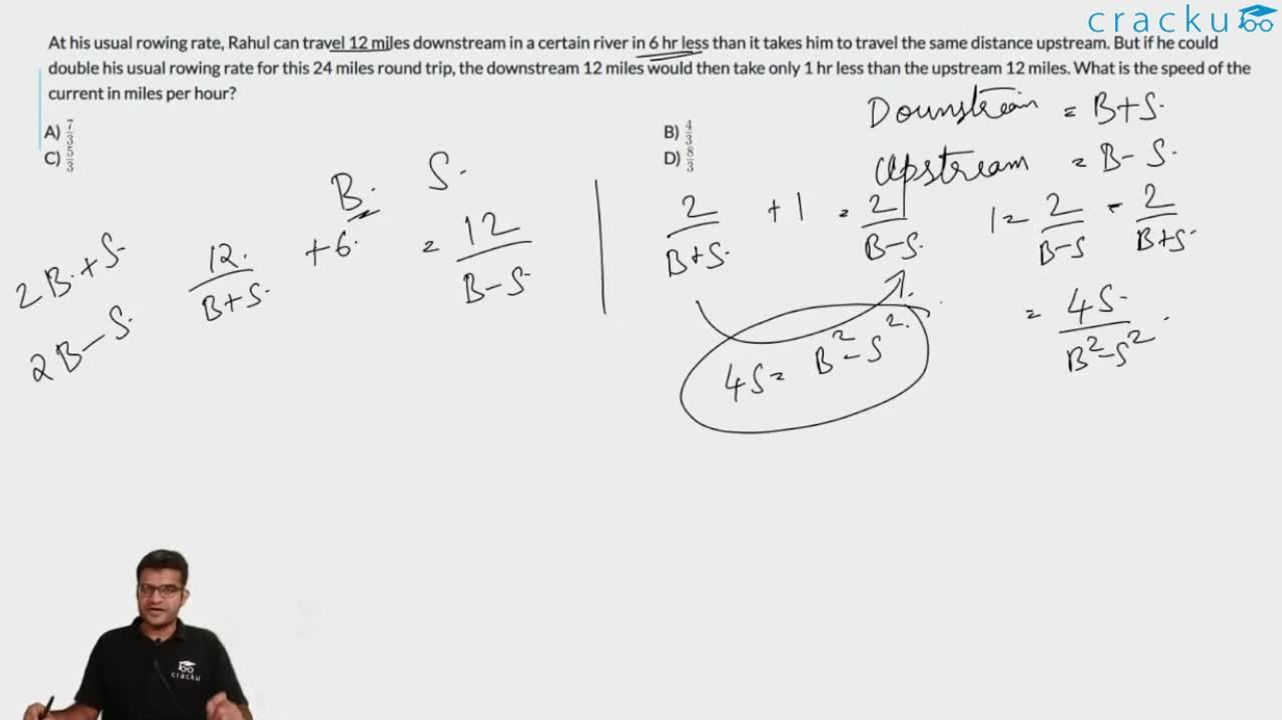Question 24

# At his usual rowing rate, Rahul can travel 12 miles downstream in a certain river in 6 hr less than it takes him to travel the same distance upstream. But if he could double his usual rowing rate for this 24 miles round trip, the downstream 12 miles would then take only 1 hr less than the upstream 12 miles. What is the speed of the current in miles per hour?

Solution

$$12/(R - S) = T$$
$$12/(R + S) = T - 6$$
$$12/(2R - S) = t$$
$$12/(2R + S) = t - 1$$
=> $$12/(R - S) - 12/(R + S) = 6$$ and $$12/(2R - S) - 12/(2R + S) = 1$$
=> $$12R + 12S - 12R + 12S = 6R^2 - 6S^2$$ and $$24R + 12S - 24R + 12S = 4R^2 - S^2$$
=> $$24S = 6R^2 - 6S^2 and 24S = 4R^2 - S^2$$
=> $$6R^2 - 6S^2 = 4R^2 - S^2$$
=> $$2R^2 = 5S^2$$
=> $$24S = 10S^2 - S^2 = 9S^2$$
=> $$S = 24/9 = 8/3$$

### View Video Solution